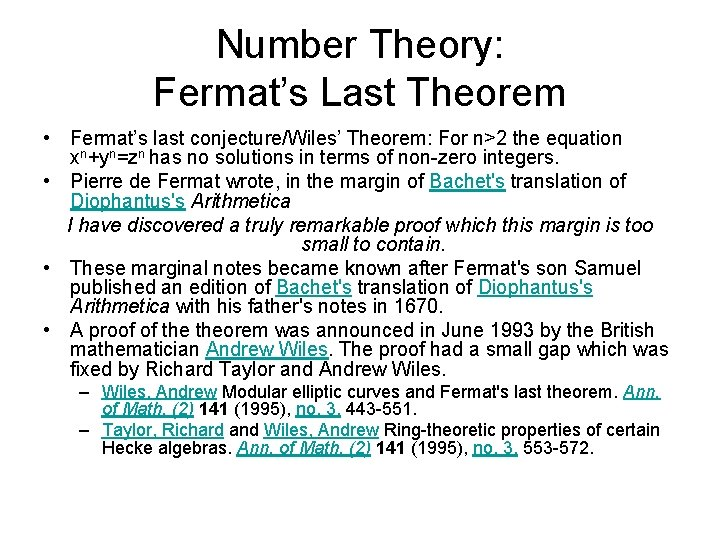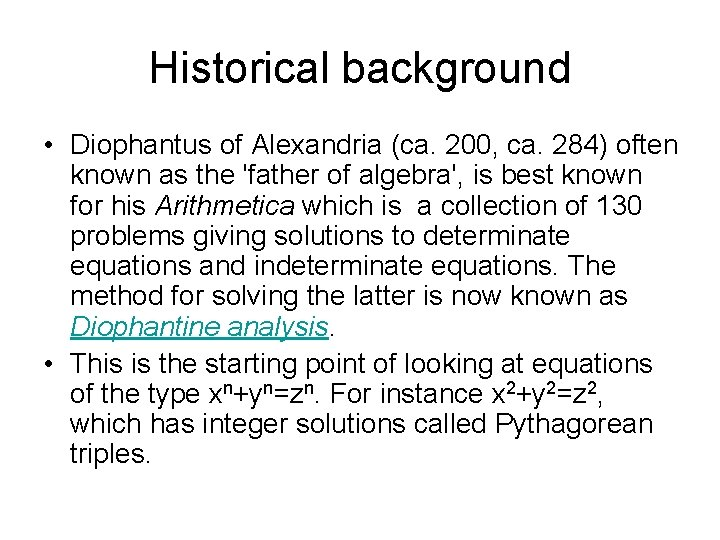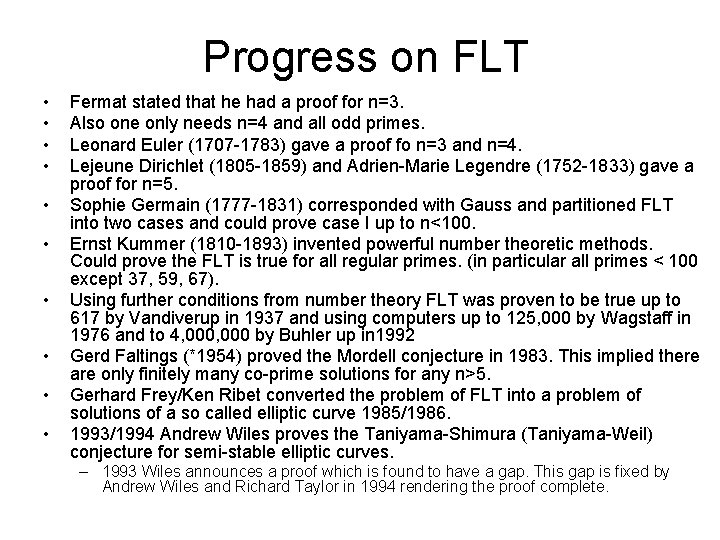# Number Theory Fermats Last Theorem Fermats last conjectureWiles

• Slides: 5
Download presentationNumber Theory: Fermat’s Last Theorem • Fermat’s last conjecture/Wiles’ Theorem: For n>2 the equation xn+yn=zn has no solutions in terms of non-zero integers. • Pierre de Fermat wrote, in the margin of Bachet's translation of Diophantus's Arithmetica I have discovered a truly remarkable proof which this margin is too small to contain. • These marginal notes became known after Fermat's son Samuel published an edition of Bachet's translation of Diophantus's Arithmetica with his father's notes in 1670. • A proof of theorem was announced in June 1993 by the British mathematician Andrew Wiles. The proof had a small gap which was fixed by Richard Taylor and Andrew Wiles. – Wiles, Andrew Modular elliptic curves and Fermat's last theorem. Ann. of Math. (2) 141 (1995), no. 3, 443 -551. – Taylor, Richard and Wiles, Andrew Ring-theoretic properties of certain Hecke algebras. Ann. of Math. (2) 141 (1995), no. 3, 553 -572.Pierre de Fermat (1601 -1665) • • • 1629 he gave a copy of his restoration of Apollonius's Plane loci 1631 received a law degree from the university Orléans. Became a government official in Toulouse and Pierre de Fermat. 1636 started correspondence with Mersenne in Paris. The typical style of Fermat's letters was that he would challenge others to find results which he had already obtained. 1653 survived the plague. Tried to correspond about number theory, but this was not the topic of the time. Results include – Method for determining Maxima and Minima and Tangents to Curved Lines caused controversy with Descartes. – Fermat's principle: light always follows the shortest possible path. – Results on number theory • Every prime of the form 4 k + 1 could be written as the sum of two squares • Fermat’s “little” Theorem. – Method of infinite descent.Historical background • Diophantus of Alexandria (ca. 200, ca. 284) often known as the 'father of algebra', is best known for his Arithmetica which is a collection of 130 problems giving solutions to determinate equations and indeterminate equations. The method for solving the latter is now known as Diophantine analysis. • This is the starting point of looking at equations of the type xn+yn=zn. For instance x 2+y 2=z 2, which has integer solutions called Pythagorean triples.Progress on FLT • • • Fermat stated that he had a proof for n=3. Also one only needs n=4 and all odd primes. Leonard Euler (1707 -1783) gave a proof fo n=3 and n=4. Lejeune Dirichlet (1805 -1859) and Adrien-Marie Legendre (1752 -1833) gave a proof for n=5. Sophie Germain (1777 -1831) corresponded with Gauss and partitioned FLT into two cases and could prove case I up to n<100. Ernst Kummer (1810 -1893) invented powerful number theoretic methods. Could prove the FLT is true for all regular primes. (in particular all primes < 100 except 37, 59, 67). Using further conditions from number theory FLT was proven to be true up to 617 by Vandiverup in 1937 and using computers up to 125, 000 by Wagstaff in 1976 and to 4, 000 by Buhler up in 1992 Gerd Faltings (*1954) proved the Mordell conjecture in 1983. This implied there are only finitely many co-prime solutions for any n>5. Gerhard Frey/Ken Ribet converted the problem of FLT into a problem of solutions of a so called elliptic curve 1985/1986. 1993/1994 Andrew Wiles proves the Taniyama-Shimura (Taniyama-Weil) conjecture for semi-stable elliptic curves. – 1993 Wiles announces a proof which is found to have a gap. This gap is fixed by Andrew Wiles and Richard Taylor in 1994 rendering the proof complete.Fermat’s little Theorem • Fermat’s Theorem: Given a prime number p and an integer a that is not divisible by p, then ap-1 has remainder 1 under division by p. Furthermore, there exists a least positive integer n such that an has remainder 1 under division by p, n divides p-1, and akn has remainder 1 under division by p for all positive integers k. • Corollary: Let p be an odd prime, and q a prime. If q divides 2 p-1, then q is of the form 2 kp + 1 for some integer k.